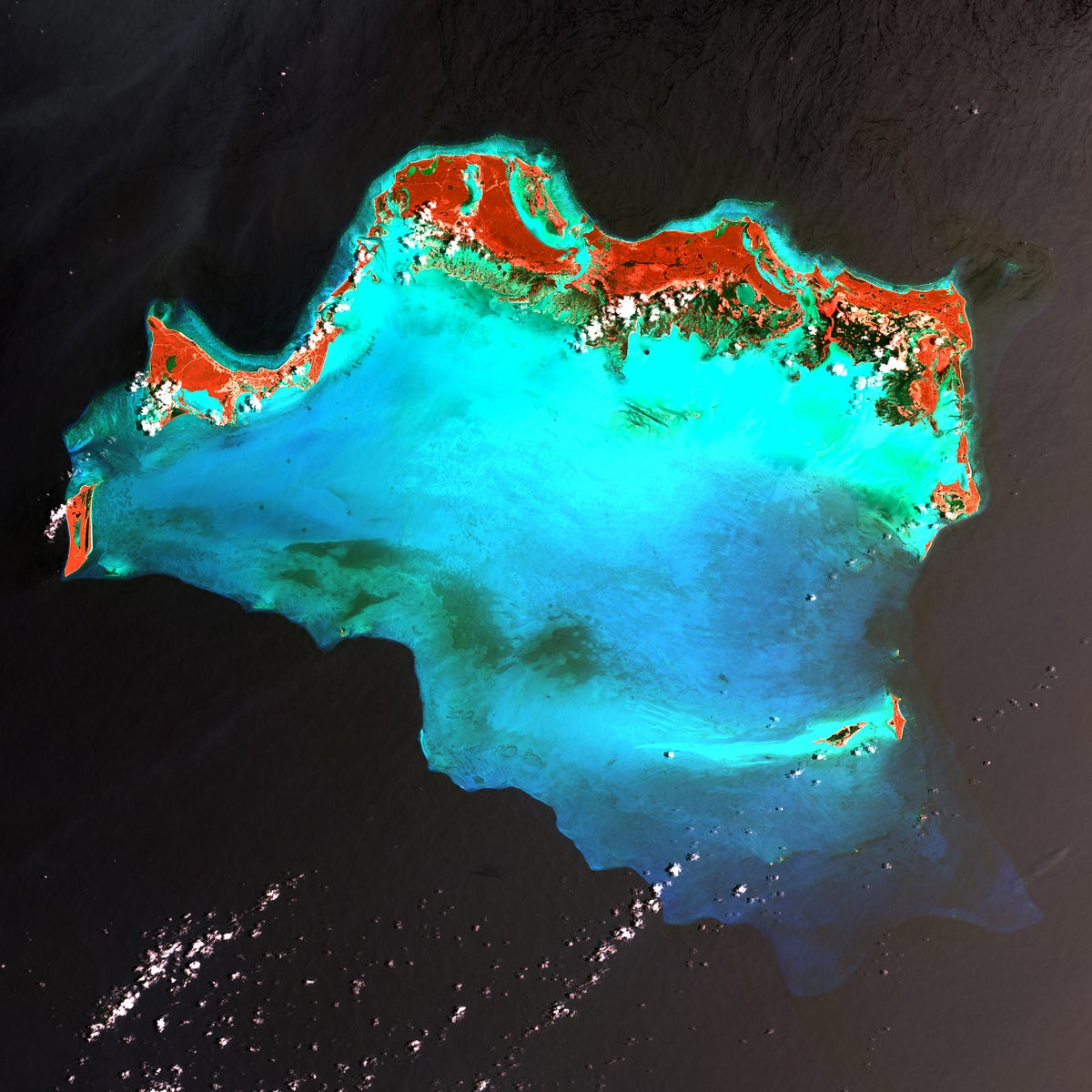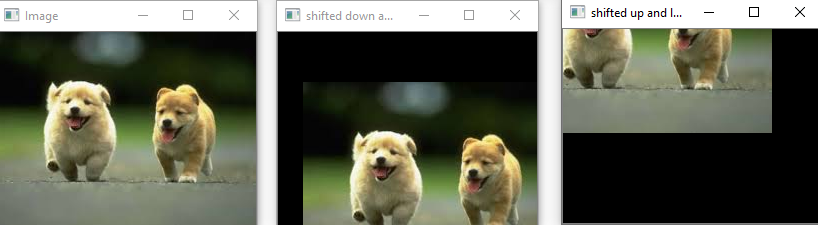# Fundamentals of OpenCV (Part-II)All the Basics of you need to know of OpenCV Library.Read further to learn how to perform image transformation using OpenCV. In Part I, I have explained what is OpenCV, and few concepts of OpenCV (How to load an image, how to read an image as NumPy array, and drawing on empty canvas). If you haven’t read the first part yet, here is the link(Fundamentals of OpenCV Part I).

Read further to learn how to perform image transformation using OpenCV.

In Part I, I have explained what is OpenCV, and few concepts of OpenCV (How to load an image, how to read an image as NumPy array, and drawing on empty canvas).

If you haven’t read the first part yet, here is the link(Fundamentals of OpenCV Part I).

In this blog, we are going to see some image transformations techniques such as translationrotationresizingflipping. So let’s begin.

Translation

Translation means the shifting of an image along the x and y-axis. Using translation, we can shift an image up, down, left, or right, in any direction we want! Below is the code showing the translation of an image.

``````import cv2
import numpy as np

cv2.imshow('Image',image)
M  = np.float32([[1,0,25],[0,1,50]])
shifted = cv2.warpAffine(image,M,(image.shape,image.shape))
cv2.imshow('shifted down and right',shifted)
M  = np.float32([[1,0,-50],[0,1,-90]])
shifted = cv2.warpAffine(image,M,(image.shape,image.shape))
cv2.imshow('shifted up and left',shifted)
cv2.waitKey(0)
cv2.destroyAllWindows()``````## Complete Guide to Mahotas Python Library for Image Processing

Processing an image in order to derive some meaningful information from the image is known as image processing. It can be called a scientific study where we apply different methods or functions on images to find out what are its different features. We can enhance the image or degrade the image in order to extract unique features.

## Python Tutorial: Image processing with Python (Using OpenCV)

In this tutorial, you will learn how you can process images in Python using the OpenCV library. It’s used to process images, videos, and even live streams, but in this tutorial, we will process images only as a first step. Before getting started, let’s install OpenCV.

## Complete Guide On Pgmagick - Python Tool For Image Processing

Complete Guide On Pgmagick - Python Tool For Image Processing. Pgmagick, open-source python library and a wrapper for GraphicsMagick, which is a collection of tools to read, write, and manipulate images

## Create a Virtual Pen and Eraser with Python OpenCV - Genial Code

Learn Free how to create a virtual pen and eraser with python and OpenCV with source code and complete guide. This entire application is built fundamentally on contour detection. It can be thought of as something like closed color curves on compromises that have the same color or intensity, it's like a blob. In this project we use color masking to get the binary mask of our target color pen, then we use the counter detection to find the location of this pen and the contour to find it.

## How to Access Pixel Data in Image using Python OpenCV

To Access Pixel Data in Image using Python OpenCV, use array shape and size property to get the width, height, channel, and pixels.